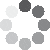Enter parameters Length & Width
Enter parameters
LENGTH, WIDTH & DEPTH
Width:
ft
• inches (in)
• feet (ft)
• yards (yd)
• centimeters (cm)
• meters (m)
Length:
ft
• inches (in)
• feet (ft)
• yards (yd)
• centimeters (cm)
• meters (m)
Price per unit of area:
ft²
• square feet (ft²)
• square inches (in²)
• square yards (yd²)
• square miles (mi²)
• acres (ac)
• square centimeters (cm²)
• square meters ()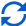Result
Total area:
0
ft²
• square feet (ft²)
• square inches (in²)
• square yards (yd²)
• square miles (mi²)
• acres (ac)
• square centimeters (cm²)
• square meters ()
Cost:
$Enter parameters Area: ft² • square feet (ft²) • square inches (in²) • square yards (yd²) • square miles (mi²) • acres (ac) • square centimeters (cm²) • square meters () Price per unit of area: ft² • square feet (ft²) • square inches (in²) • square yards (yd²) • square miles (mi²) • acres (ac) • square centimeters (cm²) • square meters ()Result Cost:$(2 votes, average: 5.00 out of 5)Loading...
Similar Calculators:
Real-time graphics. Make the calculations and see the changes.
Real-time graphics. Make the calculations and see the changes.Embed
Similar Calculators:We know how difficult home improvements can be, especially estimating how much material you will need given the size of the room.

You want to be accurate in your estimations to avoid wasting your money.

Whatever your project, you will likely need to measure the space and estimate the total cost!

That’s why we built our flooring calculator. 🔨

Use it to ensure that your next home improvement project gets off to an efficient start.

Quickly calculate the cost of the project and work out how much vinyl, laminate or hardwood flooring you’ll need to purchase.

In this article we will show you how easy the calculator is to use and how to use it.

We will also explain the Mathematical formulae it uses to work out the total area and expected cost.

Contents:

## Flooring calculator formula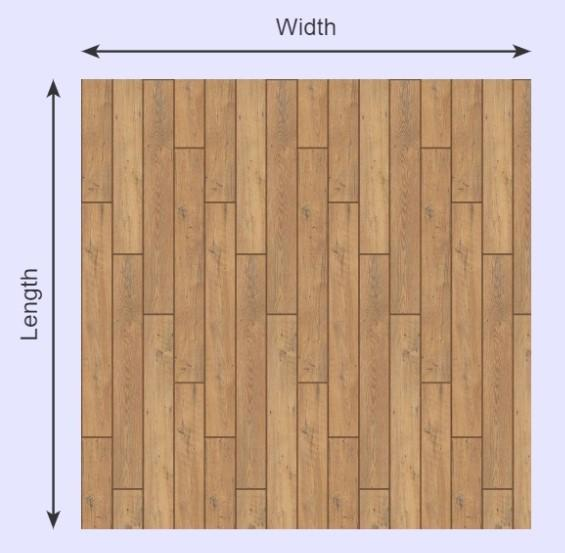Before you start using the calculator, you should measure the width and length of the room you want to lay a new floor in.

Based on these values, the calculator first calculates the area of the room in square footage.

It estimates this using the formulae:

$$Room\,Area = Length × Width$$

Once you know the room area (in square footage) you’re good to go purchase the material!

However, if you want an expected cost, you’ll need to do a second calculation:

### Calculating Total Cost of the Flooring Materials

If you know the price per unit of area (e.g. the price per square footage) of your chosen material, the calculator can work out an estimate for the total cost of the landscaping project:

$$Cost = Room\,Area × Price\,per\,Unit\,of\,Area$$

You should consider that this cost does not factor in other expenses like installation, equipment and breakage.

Obviously, if the expected cost is too high for the selected material, then you should consider using cheaper flooring material.

But, what if you don’t know the unit price?

Well, this can also easily be calculated based on the available material you purchase:

$$Price\,per\,Unit\,of\,Area = {Total\,Cost\,of\,Flooring\,Material \over Total\,Area\,of\,Material}$$

## What if I want to calculate multiple areas?

We understand that, in reality, you’re going to be flooring areas that aren’t perfectly rectangular.

It’s likely that your room (or multiple rooms!) are made up of multiple rectangles, like in our example here.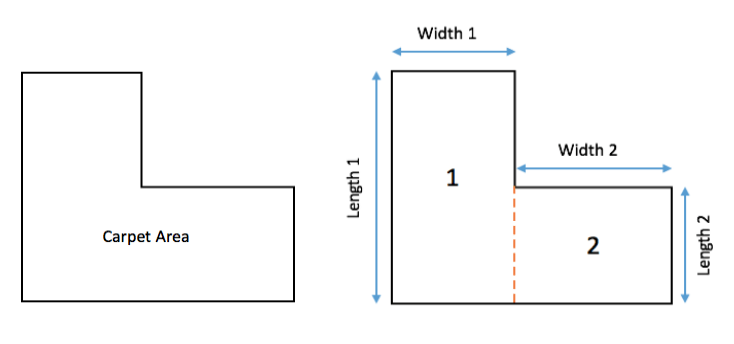But don’t worry! To figure out the total area of the room is still very simple.

All you need to do is split the total area into rectangles, calculate the area of each and then finally add these up to work out the total area:

$$Total\,Flooring\,Area = Length\,1 × Width\,1 + Length\,2 × Width\,2+⋯$$

You’ll notice that our online flooring calculator has an option for “add area”.

Use this option to calculate the total floor area if you have multiple areas or rooms by simply entering the correct values for each length and width.

Something not clear? 😓

If this doesn’t make sense, fear not!

Below, we will show you two fully worked examples starting with different initial criteria to calculate the total area, and cost of a laminated and a vinyl flooring.

Otherwise, enter your measurements and units into our flooring calculator to get your home improvement project off to an effective and efficient start!

Example Calculations

• Rectangular Laminated Flooring

Let’s imagine I want to lay down laminated floorboards in a rectangular room.

The room measures 20 feet in width and 8 feet in length.

I can purchase the laminated flooring at a cost of $4 for each square footage purchased. I want to figure out the total area of flooring and the expected total cost of the home improvement project. I would therefore enter these values and measurements into the laminate flooring calculator which would first work out the area of the room: $$Room\,Area = Length × Width = 20\,ft × 8\,ft = 160\,ft^2$$ and the expected total cost of the laminated flooring: $$Cost = Room\,Area × Price\,per\,Unit\,of\,Area = 160\,ft^2 × 4\,/ft^2 = 640$$ • Cover a more complicated layout Let’s now say I have an L-shaped room, with dimensions as shown in the picture. I want to know how much vinyl flooring I will need and how much the project will cost. I don’t know the unit price of the vinyl flooring, but I can purchase 15 square feet for$75.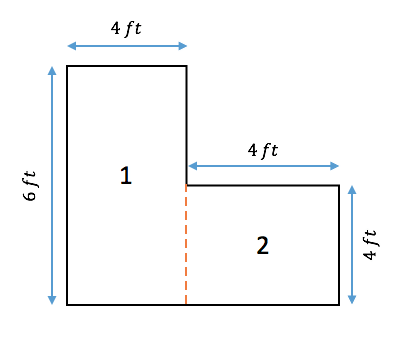I would first need to calculate the price per square footage of the vinyl flooring material:

$$Price\,per\,Unit\,of\,Area = {Total\,Cost\,of\,Flooring\,Material \over Total\,Area\,of\,Material} = {75 \over 15\,ft^2} = 5\,/ft^2$$

Based on the measurements of the room, the vinyl flooring calculator is able to work out the total area of the L-shaped room in square footage:

$$Total\,Flooring\,Area = Length\,1 × Width\,1 + Length\,2 × Width\,2 = 6\,ft × 4\,ft + 4\,ft × 4\,ft = 24\,ft^2 + 16\,ft^2 = 40\,ft^2$$

Finally, the calculator would also work out the total cost of the flooring project:

$$Cost = Room\,Area × Price\,per\,Unit\,of\,Area = 40\,ft^2 × 5\,/ft^2 = 200$$

At this point, we imagine you are wondering:

## What if my measurements aren’t in feet?

Well, the answer couldn’t be simpler. Our calculator can do all the conversions for you. 🙂

You can calculate length and width in inches, feet, yards, centimeters and meters.

Simply pick the desired units in the drop down option and the flooring calculator will do the following conversions for you:

$$1\,foot = 12\,inches = 0.33\,yards = 30.48\,centimeters = 0.3048\,meters$$

$$1\,ft^2 = 144\,in^2 = 0.111\,yd^2 = 929\,cm^2= 0.0929\,m^2$$

In addition, price per unit of area can also be given in dollars per square mile and dollars per acre.

The calculator will do the following conversions for you:

$$1\,mile^2 = 27,880,000\,ft^2$$

$$1\,acre^2 = 43,560\,ft^2$$

It’s that simple! 😉

Embed this calculator on your site!Add live graphics
Copied to clipboard! Preview